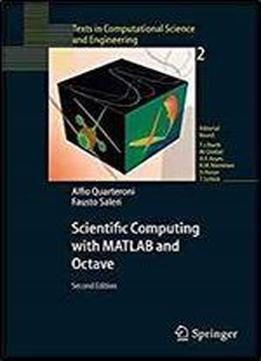# Scientific Computing With Matlab And Octave (texts In Computational Science And Engineering)Tags: Alfio Quarteroni

This textbook is an introduction to Scientific Computing, in which several numerical methods for the computer solution of certain classes of mathematical problems are illustrated. The authors show how to compute the zeros or the integrals of continuous functions, solve linear systems, approximate functions by polynomials and construct accurate approximations for the solution of ordinary and partial differential equations. To make the presentation concrete and appealing, the programming environments Matlab and Octave, which is freely distributed, are adopted as faithful companions. The book contains the solutions to several problems posed in exercises and examples, often originating from specific applications. A specific section is devoted to subjects which were not addressed in the book and contains the bibliographical references for a more comprehensive treatment of the material. The second edition features many new problems and examples, as well as more numerical methods for linear and nonlinear systems and ordinary and partial differential equations. This book is presently being translated or has appeared in the following languages: Italian, German, French, Chinese and Spanish. Reviews for "Scientific Computing with MATLAB" - 1st edition: " ... Scientific Computing with MATLAB is written in a clear and concise style, figures, tables and formula boxes complement the explanations... The whole book is an invitation, if not a request, of the authors to the reader to play with MATLAB, apply its powerful menagerie of functions to solve the given (or own) problems - in brief, supervised learning by doing .... is a stimulating introductory textbook about numerical methods that successfully combines mathematical theory with programming experience..." Anselm A.C. Horn, Journal of Molecular Modeling 2004 "... An excellent addition to academic libraries and university bookstores, this book will be useful for self-study and as a complement to other MATLAB-based books. Highly recommended. Upper-division undergraduates through professionals." S.T. Karris, Choice 2003